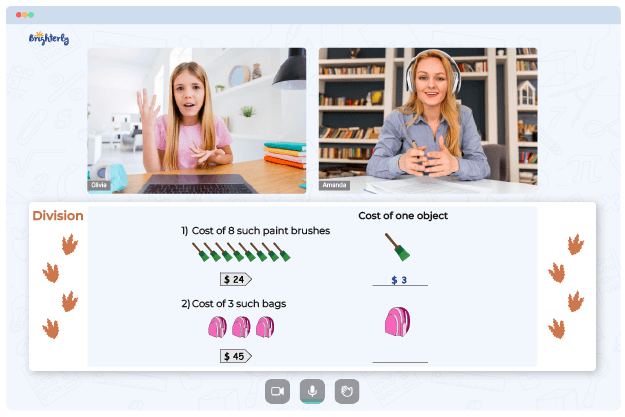# Long Division no Remainders Worksheets

The long division no remainders worksheet is used to teach or reinforce the concept of long division without remainders. The division with no remainder worksheet can be used to help students develop their long division skills, especially when dividing larger numbers.

## What Is Long Division no Remainders Worksheet?

In long division, a number (the dividend) is divided by another number (the divisor) to get the quotient and remainder. However, in this type of division without remainders worksheet, the problems are specifically designed so that the division process results in a whole number quotient with no remainder.

Math for Kids

Is Your Child Struggling With Math?
1:1 Online Math TutoringThis division no remainder worksheet contains several long division problems, each with its own dividend and divisor. The student is tasked with performing the long division algorithm, dividing the dividend by the divisor to obtain the quotient and writing the answer in the appropriate place.

You can easily download Brighterly’s long division worksheets no remainders as they are available in PDF formats.### Long Division no Remainders Worksheet PDF

Division No Remainders Worksheet### Long Division no Remainders Worksheet PDF

Division With No Remainder Worksheet### Long Division no Remainders Worksheet PDF

Division Without Remainders Worksheet### Long Division no Remainders Worksheet PDF

Long Division No Remainders Worksheet

Need help with Division?• Does your child need extra assistance with understanding division concepts?
• Start learning with an online tutor.

Is your child finding it hard to master division fundamentals? An online tutor could be of help.